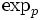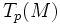# Complete equals geodesically complete

## Statement

Let$(M,g)$ be a Riemannian manifold. Then, the following are equivalent:

1.$M$ is geodesically complete: in other words, geodesics can be extended indefinitely in both directions, or equivalently, the exponential map at a point is defined on the whole tangent space at the point
2.$M$ is geodesically complete at one point: i.e. there exists$p \in M$ such that the exponential map$\exp_p$ is defined on the whole of$T_p(M)$
3.$M$ is complete as a metric space, where the distance between two points is defined as the infimum of lengths of all curves between the two points.